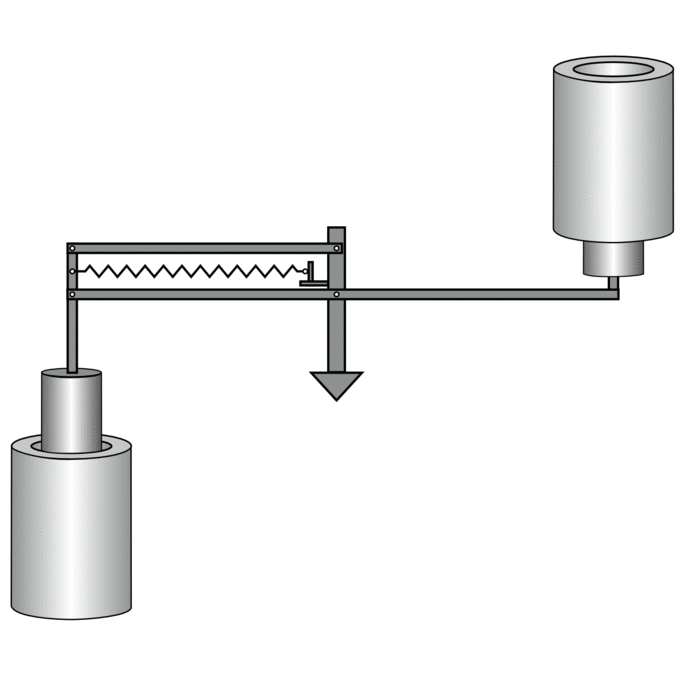A project I spent a significant amount of time on during my time in the NIST Mass and Force Group was the electrostatic force balance (EFB). The EFB is a balance which directly realises micro-Newton scale forces (or mass as local gravity at this location has been measured past the precision of the balance) using a concentric cylinder capacitor to generate an electrostatic force.

The interest in such a balance is best understood by how small mass and force metrology is performed under the current SI. Currently to get a reliable measurement of mass (or force) one must link the force to the definition of the kilogram which is defined as the mass of a lump of Platinum-Iridium in Paris. To get to down to milligram masses (microNewton forces), one must do many many comparisons measurements of the form:

This is 1 kg, these two 500 g masses equal the 1 kg (plus tiny offset plus uncertainty), these two 500 g masses equal each other (plus tiny offset plus uncertainty). These two 200 g masses and one 100 g mass equal the 500 g mass (plus tiny offset plus uncertainty) … and so on until you reach one milligram

Not only is this process tedious, the uncertainties compound, and small masses and forces have very large relative uncertainties. One suggestion was to replace the lump of Platinum-Iridium in Paris with a lump of Silicon in Germany. This lump of silicon while easier to monitor and reproduce does nothing to solve the problem of small forces. Instead the kilogram is being redefined in terms of Planck’s constant (and the lump of Silicon is helping to set that number along side Watt balances). Soon our whole system of measurements will be defined by fundamental constants of nature. After this, balances like the EFB are able measure masses and forces limited only by their own measurement uncertainty, not the uncertainty of the unit itself as they links to fundamental constants through voltages traceable to Josephson Junctions, capacitances traceable to the calculable capacitor, and an interferometer utilising the speed of light and a traceable frequency.

An animation of the EFB is provided below:This work was published in Metrologia under the title “Milligram mass metrology using an electrostatic force balance” (Open copy.)